# Quantitative Aptitude Quiz for Prelims Exams- SBI & IBPS 2020- 10th December

Directions (1-5): Table given below shows Revenue, expenditure profit and loss percentage of a company in five different years. Read the data carefully and answer the questions.
Note – Positive sign (+) shows profit percentage and negative sign ( – ) shows loss percentage.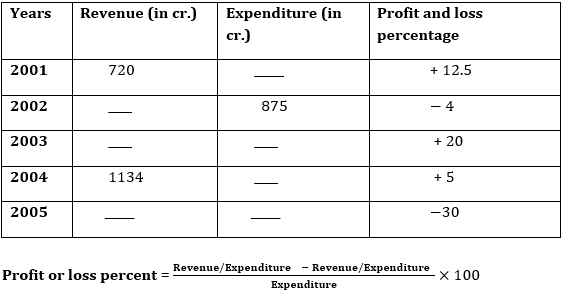Q1. Find the difference between expenditure of company in the year 2001 and revenue of company in the year 2002?
(a) 240 cr.
(b) 280 cr.
(c) 200 cr.
(d) 320 cr.
(e) 360 cr.

Q2. If profit of company in the year 2003 is two times of profit of company in the year 2001, then find the ratio of expenditure of company in the year 2001 to that of in the year 2003?
(a) 4 : 7
(b) 3 :5
(c) 4 : 9
(d) 4: 5
(e) 2 : 3

Q3. Total loss of company in the year 2002 is approximate what percent less than profit of company in the year 2004?
(a) 35%
(b) 30%
(c) 25%
(d) 20%
(e) 12%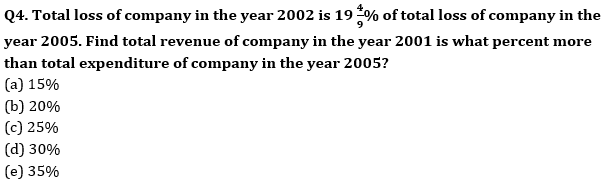Q5. If loss of company in 2005 is 180 cr. and profit in 2003 is 120cr. then find the ratio of expenditure of company in 2005 to expenditure in 2003.
(a) 1:1
(b) 1:2
(c) 2:1
(d) 3:2
(e) none of these.

Directions (6-10): Study the passage given below carefully and answer the following questions.

In a school, there are total of 243 students in 5 classes (i.e. class – I, II, III, IV & V). Students in Class – IV are 50% more than students in Class – II and students in Class – III are 10 more than students in Class – II. Students in Class – V are 80% of students in Class – IV and ratio of students in Class – I to that of in Class – V is 15 : 16.

Q6. If ratio of boys to girls in Class – I & IV is 3 : 2 and 8 : 7 respectively, then find number of girls in Class – I & IV together is what percent of total students in Class – II?
(a) 115%
(b) 130%
(c) 120%
(d) 135%
(e) 125%

Q7. If ratio of students who play basketball, football and cricket in Class – III & V is 2 : 1 : 2 and 2 : 3 : 3 respectively, then find ratio of students who play football in these 2 classes together to students who play cricket in these two classes together.
(a) 11 : 9
(b) 1 : 1
(c) 7 : 4
(d) 6 : 1
(e) None of the above.

Q8. If ratio of girls to boys in Class – II is 2 : 3 and average weight of boys in Class – II is 40kg and average weight of girls in Class – II is 25kg, then find the average weight of Class – II.
(a) 33 kg
(b) 34 kg
(c) 37 kg
(d) 36 kg
(e) 35 kg

Q9. Find average number of students in Class – II, III & V.
(a) 52
(b) 46
(c) 45
(d) 42
(e) 49

Q10. If total students in Class – VI are equal to 150% of average number of students in Class – II & V, then find difference between total students in Class – VI and total students in Class – IV.
(a) 18
(b) 9
(c) 12
(d) 6
(e) 15

Directions (11-15): Read the given information carefully and answer the following questions.
A, B, C and D started a business in partnership. A invested Rs. 5000 for whole period whereas the average of initial investment for all four is Rs. 5975. Investment made by D is 25% more than B and ratio of investment made by B to that of C is 10 : 9. Time period for which B invested his amount is 2/3rd of that of A. Sum of time period for which C & D invested is equal to total time period for which A & B invested and profit share of C is 18/79th of total profit at the end of the year.

Q11. Find ratio of period of investment of C to that of D?
(a) 1 : 1
(b) 1 : 2
(c) 2 : 3
(d) 3 : 2
(e) 4 : 3

Q12. Difference between investment of D & C is what percent of difference between investment of B & D?
(a) 125%
(b) 100%
(c) 150%
(d) 140%
(e) 160%

Q13. If at the end of business term, total profit is Rs. 15,800, then find the difference between profit share of A & D.
(a) Rs. 800
(b) Rs. 1200
(c) Rs. 1000
(d) Rs. 1500
(e) None of these

Q14. Profit share of B is what percent of profit share of A?
(a) 80%
(b) 75%
(c) 60%
(d) 100%
(e) 65%

Q15. Investment of C & D together is approximately what percent of total investment of all the four?
(a) 45%
(b) 48%
(c) 50%
(d) 52%
(e) 54%

Practice More Questions of Quantitative Aptitude for Competitive Exams:

Solutions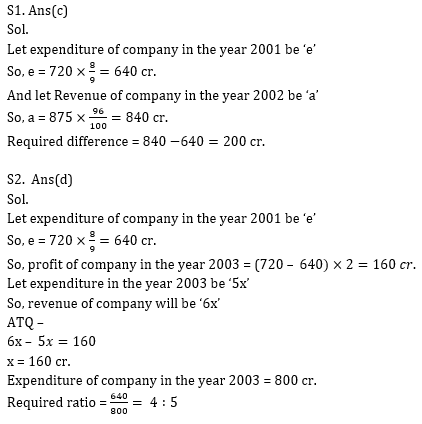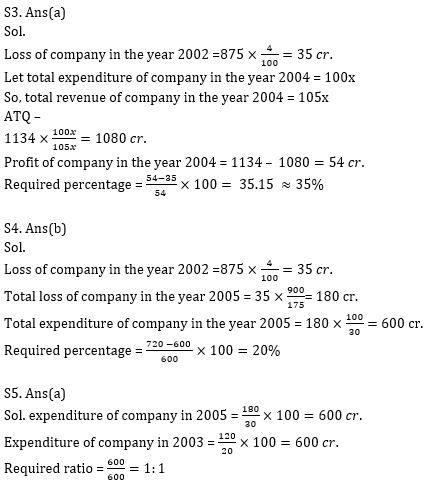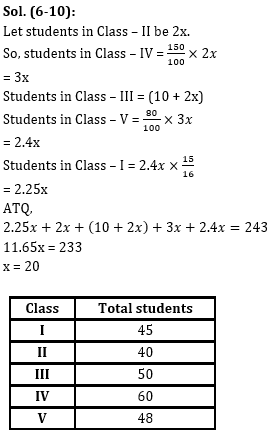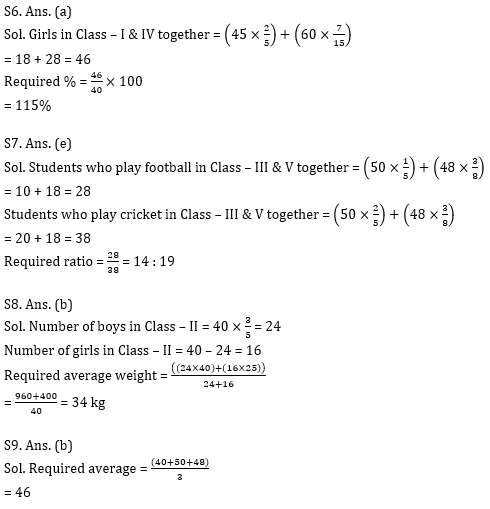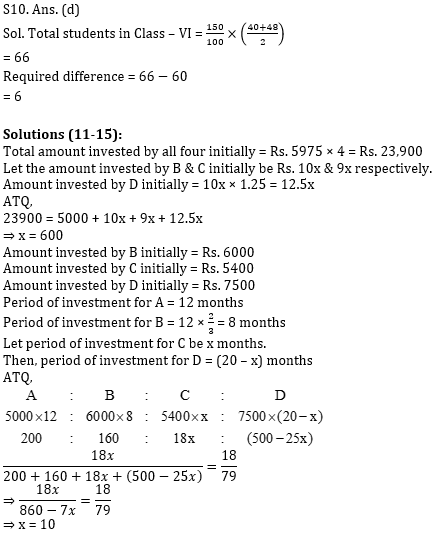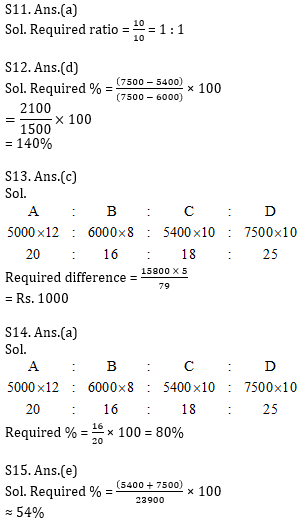Practice with Crash Course and Online Test Series for IBPS Clerk Prelims: